Reconstruction of the 2016 Ranscomb Bottom formation 1.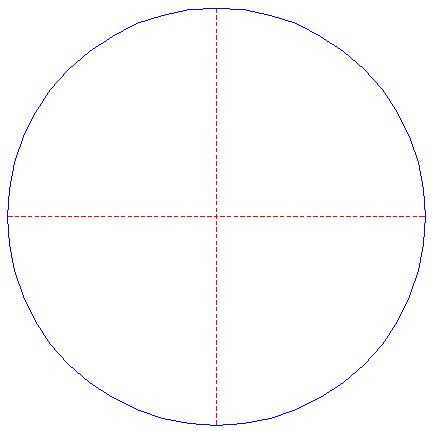Draw a circle. Draw the horizontal and vertical centerlines. 2.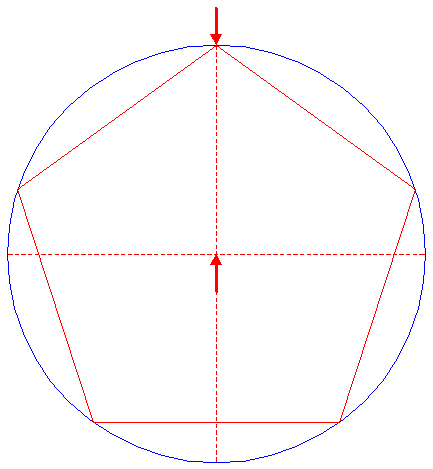Construct the inscribed pentagon (regular 5-sided polygon) of circle 1, pointing up. 3.Draw the five diagonals of pentagon 2. Number these diagonals clockwise 1 - 5, starting at the horizontal one. 4.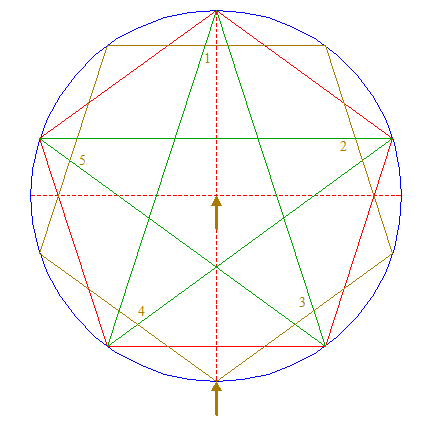Construct the inscribed pentagon of circle 1, pointing down. Number the sides clockwise 1 - 5, starting at the upper one. 5.Construct the circumscribed pentagon of circle 1, the lower side horizontally. 6.Extend the sides of pentagon 2 each in both directions upto pentagon 5. 7.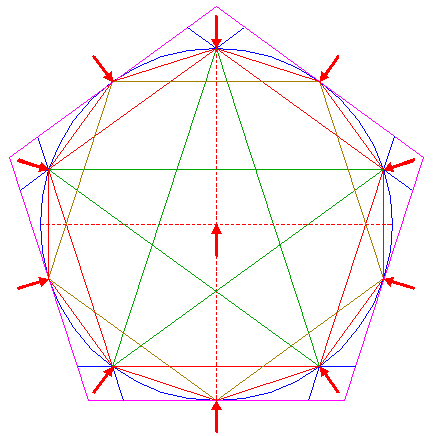Construct the inscribed decagon (regular 10-sided polygon) of circle 1, by connecting the successive angular points of pentagons 2 and 5. 8.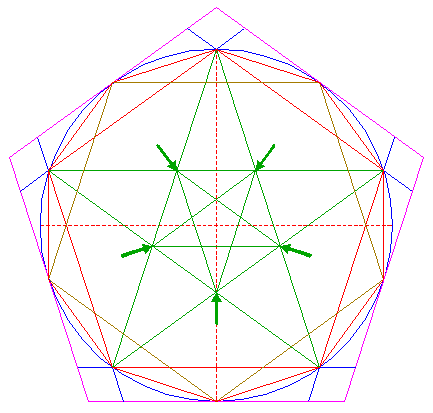Draw the five diagonals of the pentagon enclosed by diagonals 3. 9.Draw the five diagonals of pentagon 4. 10.Draw the connecting line between the intersection of diagonal 3 nr. 5 and side 4 nr. 1 and the intersection of diagonal 3 nr. 1 and side 4 nr. 5. Repeat this four times for the other corresponding intersections of diagonals 3 and sides 4, as shown. 11.Draw the two diagonals of the lower righthand parallelogram enclosed by pentagon 5 and lines 6. 12.Construct a "two-points" circle (defined by the two end-points of a centerline) between the intersection of the upper lefthand and upper righthand diagonals 9 and the intersection of diagonal 3 nr. 1 and the vertical centerline. 13.Copy circle 12 to the intersection of diagonals 11. 14.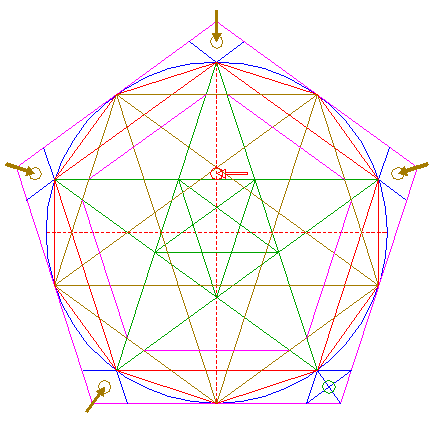Copy circle 12 four more times to the other positions with respect to pentagon 5 corresponding to circle 13, as shown. 15.Lines 3, 4, 5, 6, 7, 8, 9, and 10, and circles 13 and 14, are used for the final reconstruction. 16.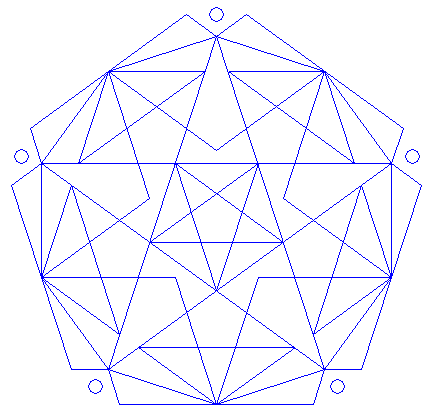Remove all parts not visible within the formation itself. 17.Colour all areas corresponding to standing... 18.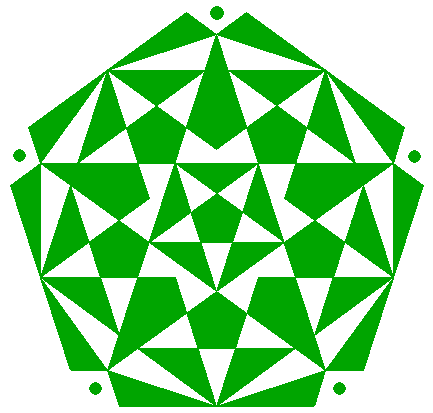...or to flattened crop, and finish the reconstruction of the 2016 Ranscomb Bottom formation. 19.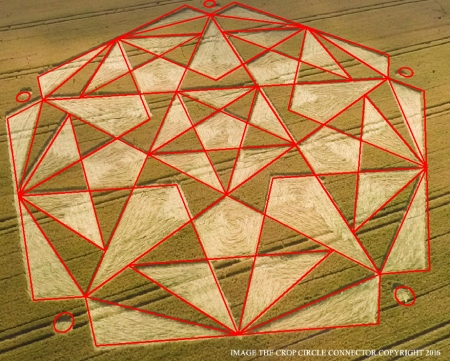The final result, matched with two aerial images.